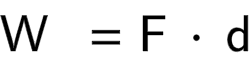# Work Calculator

Created by Dominik Czernia, PhD
Last updated: Jul 01, 2022

Use our work calculator to quickly estimate the work in physics based on two different methods. In our tool, we added two formulae for work:

1. The simplest work = force × distance expression; and
2. The velocity change equation based on the formula for kinetic energy.

The following article explains how to find work in physics, what work units are, and how to calculate work done with two approaches.

If you're looking for the thermodynamic definition and application of work, check the combined gas law calculator, where we describe various processes for many types of gases.

## How to find work in physics

The energy of an object can alter essentially in two ways: by work or heat. There is no single work formula in physics. That's because we define this quantity in classical mechanics for moving objects and in thermodynamics for processes involving volume change. In this work calculator, we focus on the former meaning.

So, what is the formula for work? The first work equation you will ever see during physics classes is probably the work = force × distance expression. So, you simply multiply the force acting on an object by the distance the object was moved by due to the force. We say this is the work done formula, in particular, done by external force if an object hasn't moved by itself. The SI unit of work are joules, abbreviated as J.

⚠️ A more formal and general way to calculate work is by using integrals, but we won't go into details because this is out of the scope of this tool. You need to use it, e.g., to find the potential energy of a charge that is subjected to the electrostatic force. Check the article about the Coulomb's law equation to learn more regarding the force between charges.

## What is the formula for work using velocities?

Another work equation results from the difference between the initial and final kinetic energies:

$W = E_1 - E_0 = \frac{1}{2}m(v_1^2 - v_0^2)$

where:

• $W$ - The work done on the object (by work definition in physics, the SI unit of work is a joule);
• $E_1$ and $E_0$ - The final and initial kinetic energies of an object;
• $m$ - The mass of the object; and
• $v_1$ and $v_0$ - The final and initial velocities of an object.

## How to use the work calculator

Let's see how to calculate work on a specific example:

1. You have moved a box by 5 feet (1.5 m) using the force of 70 N. This is how to calculate work done.
2. Use the first work done formula, namely: force and displacement.
3. Enter the appropriate values into the work calculator.
4. Read the final result: work equals approximately 107 J in that case.

That's it! We hope the work definition in physics will not confuse you anymore.

Dominik Czernia, PhDCalculate from
force and displacement
Work calculation
Force (F)
N
Displacement (d)
yd
Work (W)
J
People also viewed…

### Arrow speed

Arrow speed calculator finds the real speed of an arrow for a bow with custom parameters.